# Selina Solutions Concise Maths Class 7 Chapter 3: Fraction (Including Problems) Exercise 3B

Selina Solutions Concise Maths Class 7 Chapter 3 Fraction (Including Problems) Exercise 3B covers concepts like unlike and like fractions and their conversion using the steps mentioned in the textbook. The steps to be followed in their conversion are explained in simple language, in order to make the subject interesting among students. By practising these textbook problems on a regular basis, students can improve their problem solving abilities. Selina Solutions Concise Maths Class 7 Chapter 3 Fraction (Including Problems) Exercise 3B PDF link is given below, with a free download option.

## Selina Solutions Concise Maths Class 7 Chapter 3: Fraction (Including Problems) Exercise 3B Download PDF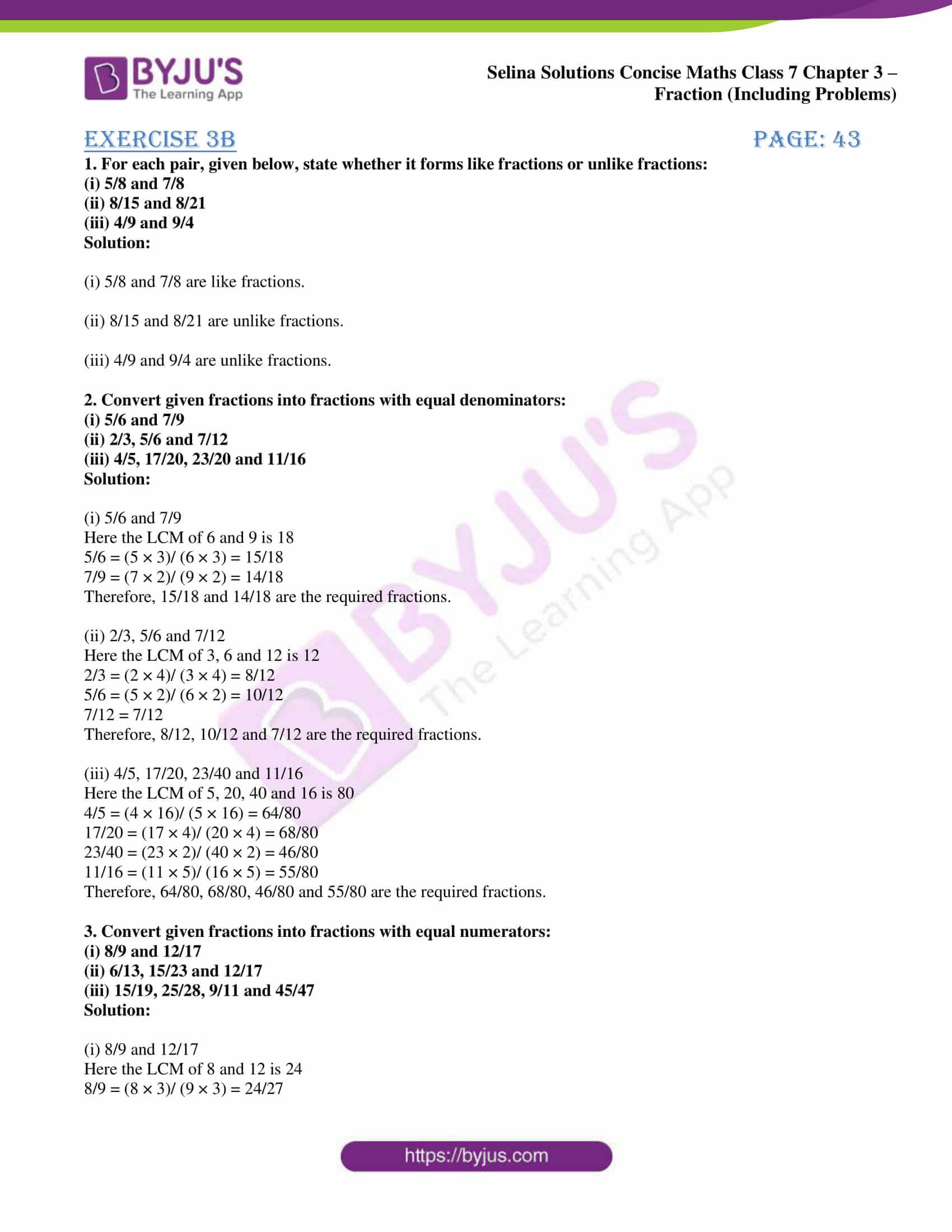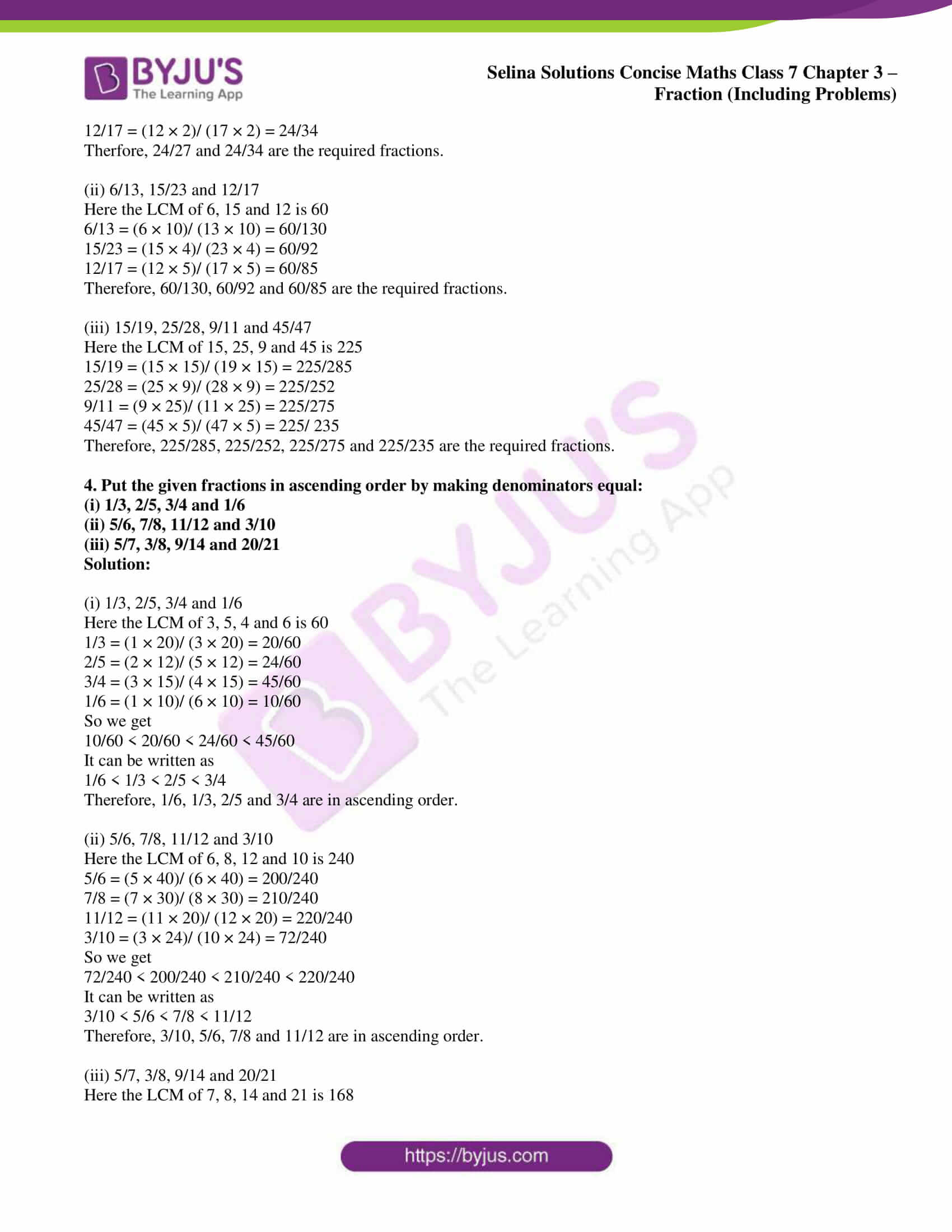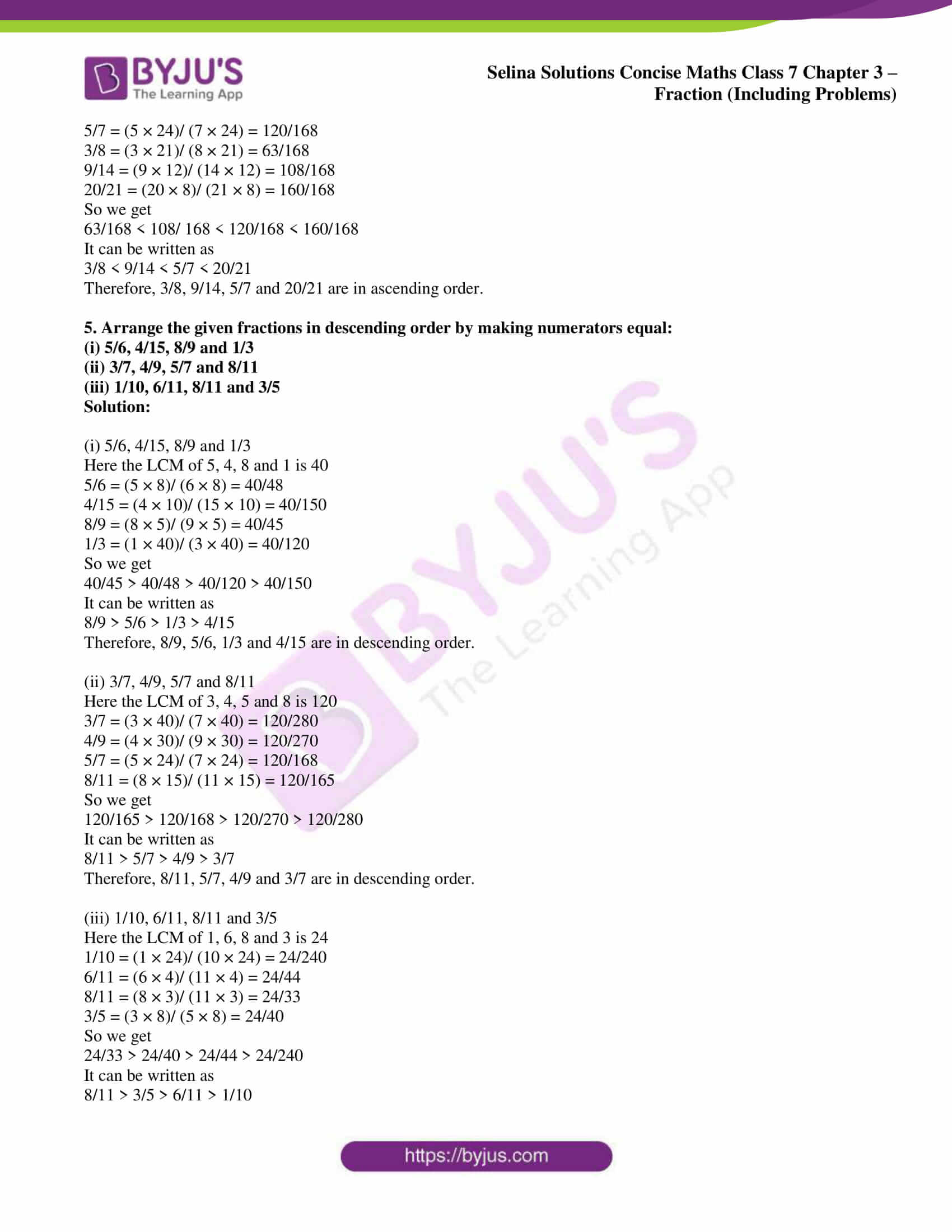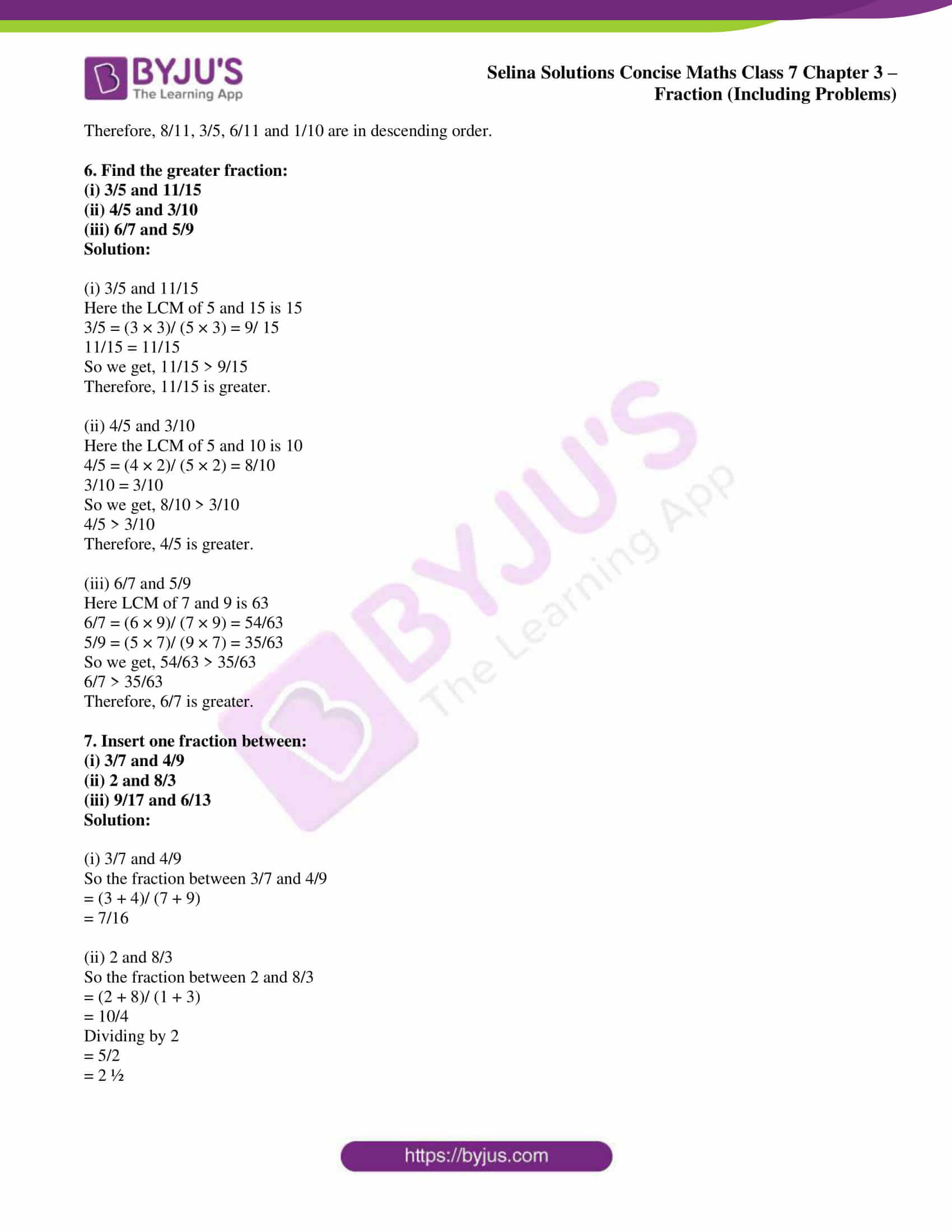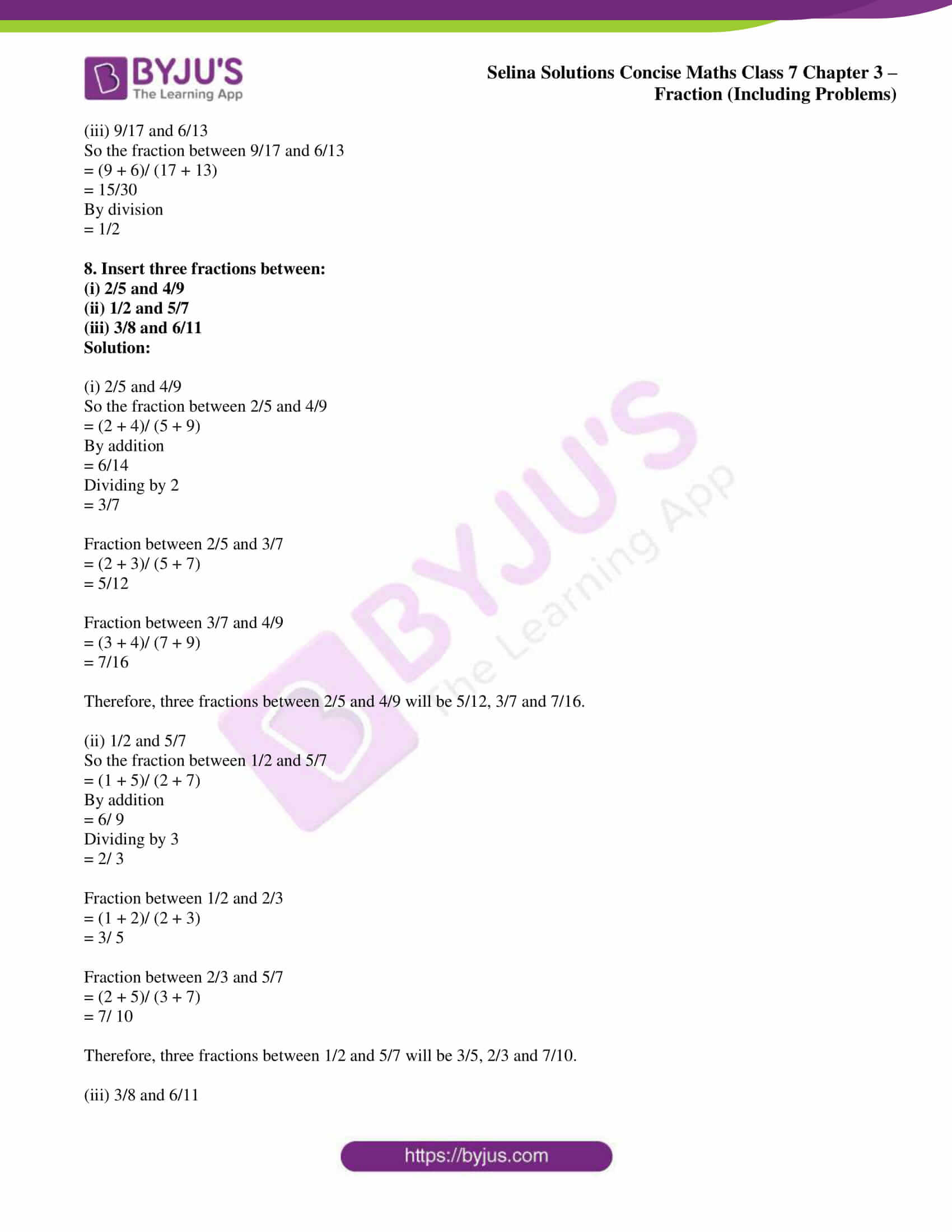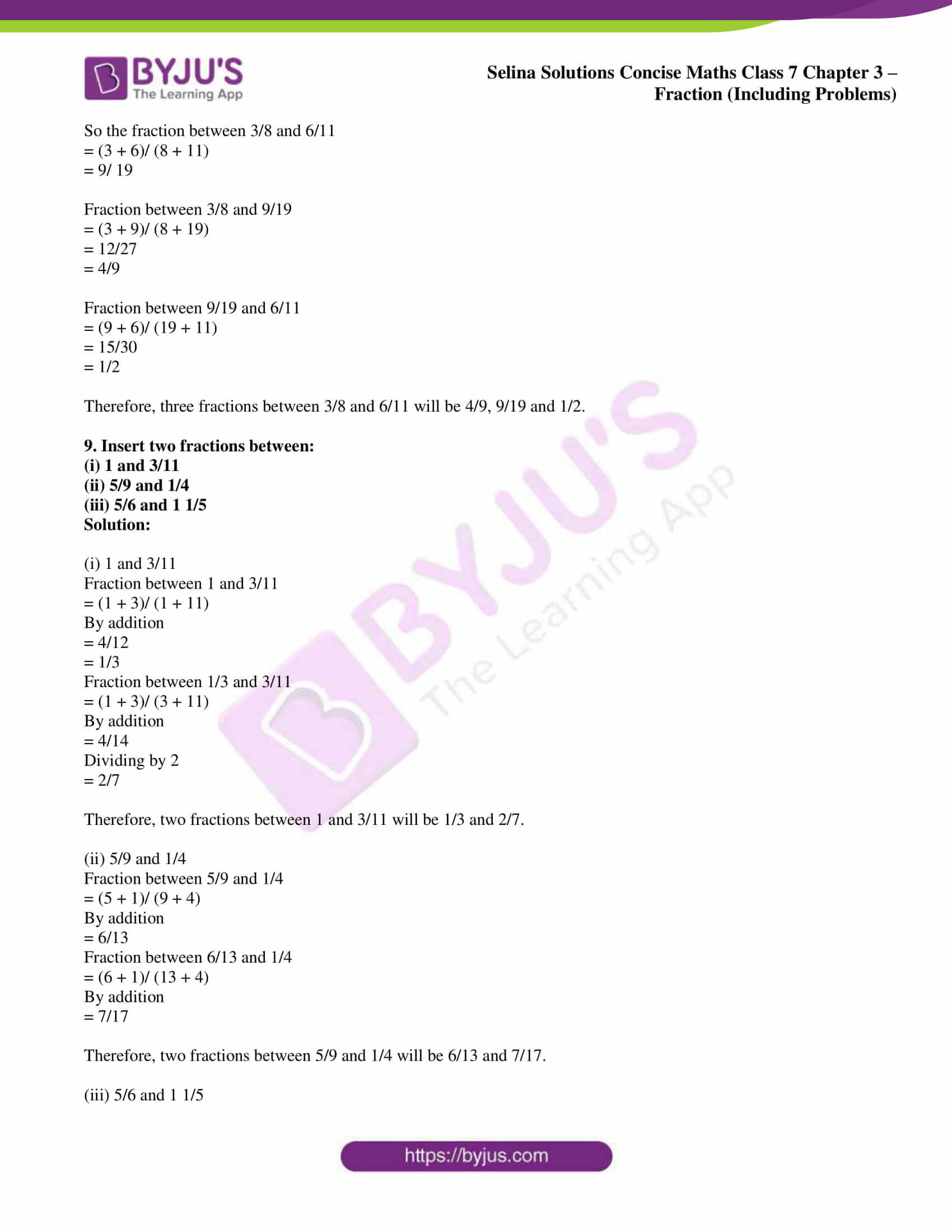### Access Selina Solutions Concise Maths Class 7 Chapter 3: Fraction (Including Problems) Exercise 3B

1. For each pair, given below, state whether it forms like fractions or unlike fractions:

(i) 5/8 and 7/8

(ii) 8/15 and 8/21

(iii) 4/9 and 9/4

Solution:

(i) 5/8 and 7/8 are like fractions.

(ii) 8/15 and 8/21 are unlike fractions.

(iii) 4/9 and 9/4 are unlike fractions.

2. Convert given fractions into fractions with equal denominators:

(i) 5/6 and 7/9

(ii) 2/3, 5/6 and 7/12

(iii) 4/5, 17/20, 23/20 and 11/16

Solution:

(i) 5/6 and 7/9

Here the LCM of 6 and 9 is 18

5/6 = (5 × 3)/ (6 × 3) = 15/18

7/9 = (7 × 2)/ (9 × 2) = 14/18

Therefore, 15/18 and 14/18 are the required fractions.

(ii) 2/3, 5/6 and 7/12

Here the LCM of 3, 6 and 12 is 12

2/3 = (2 × 4)/ (3 × 4) = 8/12

5/6 = (5 × 2)/ (6 × 2) = 10/12

7/12 = 7/12

Therefore, 8/12, 10/12 and 7/12 are the required fractions.

(iii) 4/5, 17/20, 23/40 and 11/16

Here the LCM of 5, 20, 40 and 16 is 80

4/5 = (4 × 16)/ (5 × 16) = 64/80

17/20 = (17 × 4)/ (20 × 4) = 68/80

23/40 = (23 × 2)/ (40 × 2) = 46/80

11/16 = (11 × 5)/ (16 × 5) = 55/80

Therefore, 64/80, 68/80, 46/80 and 55/80 are the required fractions.

3. Convert given fractions into fractions with equal numerators:

(i) 8/9 and 12/17

(ii) 6/13, 15/23 and 12/17

(iii) 15/19, 25/28, 9/11 and 45/47

Solution:

(i) 8/9 and 12/17

Here the LCM of 8 and 12 is 24

8/9 = (8 × 3)/ (9 × 3) = 24/27

12/17 = (12 × 2)/ (17 × 2) = 24/34

Therfore, 24/27 and 24/34 are the required fractions.

(ii) 6/13, 15/23 and 12/17

Here the LCM of 6, 15 and 12 is 60

6/13 = (6 × 10)/ (13 × 10) = 60/130

15/23 = (15 × 4)/ (23 × 4) = 60/92

12/17 = (12 × 5)/ (17 × 5) = 60/85

Therefore, 60/130, 60/92 and 60/85 are the required fractions.

(iii) 15/19, 25/28, 9/11 and 45/47

Here the LCM of 15, 25, 9 and 45 is 225

15/19 = (15 × 15)/ (19 × 15) = 225/285

25/28 = (25 × 9)/ (28 × 9) = 225/252

9/11 = (9 × 25)/ (11 × 25) = 225/275

45/47 = (45 × 5)/ (47 × 5) = 225/ 235

Therefore, 225/285, 225/252, 225/275 and 225/235 are the required fractions.

4. Put the given fractions in ascending order by making denominators equal:

(i) 1/3, 2/5, 3/4 and 1/6

(ii) 5/6, 7/8, 11/12 and 3/10

(iii) 5/7, 3/8, 9/14 and 20/21

Solution:

(i) 1/3, 2/5, 3/4 and 1/6

Here the LCM of 3, 5, 4 and 6 is 60

1/3 = (1 × 20)/ (3 × 20) = 20/60

2/5 = (2 × 12)/ (5 × 12) = 24/60

3/4 = (3 × 15)/ (4 × 15) = 45/60

1/6 = (1 × 10)/ (6 × 10) = 10/60

So we get

10/60 < 20/60 < 24/60 < 45/60

It can be written as

1/6 < 1/3 < 2/5 < 3/4

Therefore, 1/6, 1/3, 2/5 and 3/4 are in ascending order.

(ii) 5/6, 7/8, 11/12 and 3/10

Here the LCM of 6, 8, 12 and 10 is 240

5/6 = (5 × 40)/ (6 × 40) = 200/240

7/8 = (7 × 30)/ (8 × 30) = 210/240

11/12 = (11 × 20)/ (12 × 20) = 220/240

3/10 = (3 × 24)/ (10 × 24) = 72/240

So we get

72/240 < 200/240 < 210/240 < 220/240

It can be written as

3/10 < 5/6 < 7/8 < 11/12

Therefore, 3/10, 5/6, 7/8 and 11/12 are in ascending order.

(iii) 5/7, 3/8, 9/14 and 20/21

Here the LCM of 7, 8, 14 and 21 is 168

5/7 = (5 × 24)/ (7 × 24) = 120/168

3/8 = (3 × 21)/ (8 × 21) = 63/168

9/14 = (9 × 12)/ (14 × 12) = 108/168

20/21 = (20 × 8)/ (21 × 8) = 160/168

So we get

63/168 < 108/ 168 < 120/168 < 160/168

It can be written as

3/8 < 9/14 < 5/7 < 20/21

Therefore, 3/8, 9/14, 5/7 and 20/21 are in ascending order.

5. Arrange the given fractions in descending order by making numerators equal:

(i) 5/6, 4/15, 8/9 and 1/3

(ii) 3/7, 4/9, 5/7 and 8/11

(iii) 1/10, 6/11, 8/11 and 3/5

Solution:

(i) 5/6, 4/15, 8/9 and 1/3

Here the LCM of 5, 4, 8 and 1 is 40

5/6 = (5 × 8)/ (6 × 8) = 40/48

4/15 = (4 × 10)/ (15 × 10) = 40/150

8/9 = (8 × 5)/ (9 × 5) = 40/45

1/3 = (1 × 40)/ (3 × 40) = 40/120

So we get

40/45 > 40/48 > 40/120 > 40/150

It can be written as

8/9 > 5/6 > 1/3 > 4/15

Therefore, 8/9, 5/6, 1/3 and 4/15 are in descending order.

(ii) 3/7, 4/9, 5/7 and 8/11

Here the LCM of 3, 4, 5 and 8 is 120

3/7 = (3 × 40)/ (7 × 40) = 120/280

4/9 = (4 × 30)/ (9 × 30) = 120/270

5/7 = (5 × 24)/ (7 × 24) = 120/168

8/11 = (8 × 15)/ (11 × 15) = 120/165

So we get

120/165 > 120/168 > 120/270 > 120/280

It can be written as

8/11 > 5/7 > 4/9 > 3/7

Therefore, 8/11, 5/7, 4/9 and 3/7 are in descending order.

(iii) 1/10, 6/11, 8/11 and 3/5

Here the LCM of 1, 6, 8 and 3 is 24

1/10 = (1 × 24)/ (10 × 24) = 24/240

6/11 = (6 × 4)/ (11 × 4) = 24/44

8/11 = (8 × 3)/ (11 × 3) = 24/33

3/5 = (3 × 8)/ (5 × 8) = 24/40

So we get

24/33 > 24/40 > 24/44 > 24/240

It can be written as

8/11 > 3/5 > 6/11 > 1/10

Therefore, 8/11, 3/5, 6/11 and 1/10 are in descending order.

6. Find the greater fraction:

(i) 3/5 and 11/15

(ii) 4/5 and 3/10

(iii) 6/7 and 5/9

Solution:

(i) 3/5 and 11/15

Here the LCM of 5 and 15 is 15

3/5 = (3 × 3)/ (5 × 3) = 9/ 15

11/15 = 11/15

So we get, 11/15 > 9/15

Therefore, 11/15 is greater.

(ii) 4/5 and 3/10

Here the LCM of 5 and 10 is 10

4/5 = (4 × 2)/ (5 × 2) = 8/10

3/10 = 3/10

So we get, 8/10 > 3/10

4/5 > 3/10

Therefore, 4/5 is greater.

(iii) 6/7 and 5/9

Here LCM of 7 and 9 is 63

6/7 = (6 × 9)/ (7 × 9) = 54/63

5/9 = (5 × 7)/ (9 × 7) = 35/63

So we get, 54/63 > 35/63

6/7 > 35/63

Therefore, 6/7 is greater.

7. Insert one fraction between:
(i) 3/7 and 4/9

(ii) 2 and 8/3

(iii) 9/17 and 6/13

Solution:

(i) 3/7 and 4/9

So the fraction between 3/7 and 4/9

= (3 + 4)/ (7 + 9)

= 7/16

(ii) 2 and 8/3

So the fraction between 2 and 8/3

= (2 + 8)/ (1 + 3)

= 10/4

Dividing by 2

= 5/2

= 2 ½

(iii) 9/17 and 6/13

So the fraction between 9/17 and 6/13

= (9 + 6)/ (17 + 13)

= 15/30

By division

= 1/2

8. Insert three fractions between:

(i) 2/5 and 4/9

(ii) 1/2 and 5/7

(iii) 3/8 and 6/11

Solution:

(i) 2/5 and 4/9

So the fraction between 2/5 and 4/9

= (2 + 4)/ (5 + 9)

= 6/14

Dividing by 2

= 3/7

Fraction between 2/5 and 3/7

= (2 + 3)/ (5 + 7)

= 5/12

Fraction between 3/7 and 4/9

= (3 + 4)/ (7 + 9)

= 7/16

Therefore, three fractions between 2/5 and 4/9 will be 5/12, 3/7 and 7/16.

(ii) 1/2 and 5/7

So the fraction between 1/2 and 5/7

= (1 + 5)/ (2 + 7)

= 6/ 9

Dividing by 3

= 2/ 3

Fraction between 1/2 and 2/3

= (1 + 2)/ (2 + 3)

= 3/ 5

Fraction between 2/3 and 5/7

= (2 + 5)/ (3 + 7)

= 7/ 10

Therefore, three fractions between 1/2 and 5/7 will be 3/5, 2/3 and 7/10.

(iii) 3/8 and 6/11

So the fraction between 3/8 and 6/11

= (3 + 6)/ (8 + 11)

= 9/ 19

Fraction between 3/8 and 9/19

= (3 + 9)/ (8 + 19)

= 12/27

= 4/9

Fraction between 9/19 and 6/11

= (9 + 6)/ (19 + 11)

= 15/30

= 1/2

Therefore, three fractions between 3/8 and 6/11 will be 4/9, 9/19 and 1/2.

9. Insert two fractions between:

(i) 1 and 3/11

(ii) 5/9 and 1/4

(iii) 5/6 and 1 1/5

Solution:

(i) 1 and 3/11

Fraction between 1 and 3/11

= (1 + 3)/ (1 + 11)

= 4/12

= 1/3

Fraction between 1/3 and 3/11

= (1 + 3)/ (3 + 11)

= 4/14

Dividing by 2

= 2/7

Therefore, two fractions between 1 and 3/11 will be 1/3 and 2/7.

(ii) 5/9 and 1/4

Fraction between 5/9 and 1/4

= (5 + 1)/ (9 + 4)

= 6/13

Fraction between 6/13 and 1/4

= (6 + 1)/ (13 + 4)

= 7/17

Therefore, two fractions between 5/9 and 1/4 will be 6/13 and 7/17.

(iii) 5/6 and 1 1/5

It can be written as

= 5/6 + 6/5

Fraction between 5/6 and 6/5

= (5 + 6)/ (6 + 5)

= 11/11

= 1

Fraction between 1 and 6/5

= (1 + 6)/ (1 + 5)